# Blog Archives

## Using clustering to find points in an image

November 26, 2018
By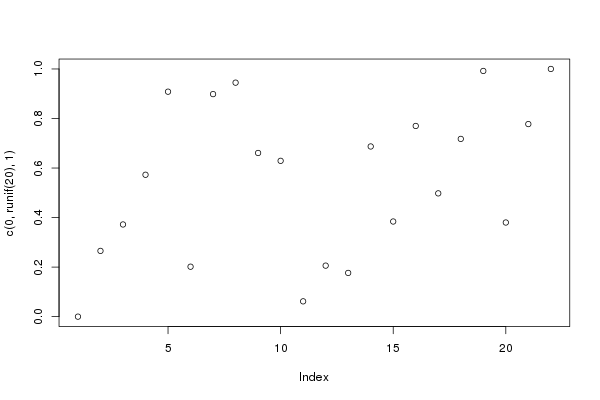In this post, I present my new package {img2coord}. This package can be used to retrieve coordinates from a scatter plot (as an image). devtools::install_github("privefl/img2coord") Have you ever made a plot, saved it as a png and moved on? When you come back to it, it is sometimes difficult to read the values from this plot, especially if there...

## Choosing hyper-parameters in penalized regression

November 22, 2018
By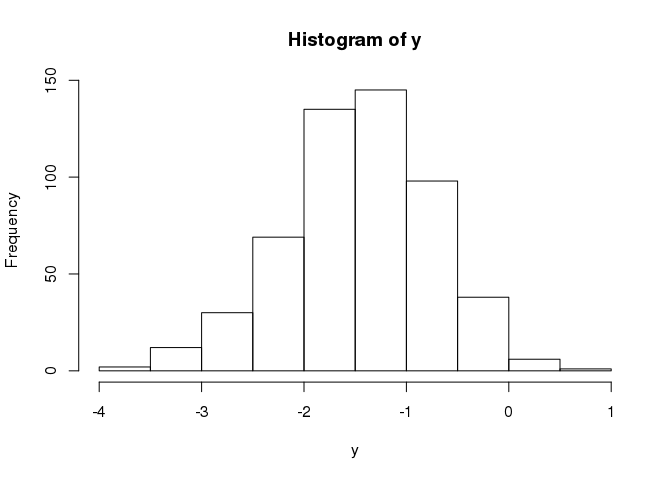In this post, I’m evaluating some ways of choosing hyper-parameters ($$\alpha$$ and $$\lambda$$) in penalized linear regression. The same principles can be applied to other types of penalized regresions (e.g. logistic). Model In penalized linear regression, we find regression coefficients $$\hat{\beta}_0$$ and $$\hat{\beta}$$ that minimize the following regularized loss function \[L(\lambda, \alpha) = \underbrace{ \frac{1}{2n} \sum_{i=1}^n \left( y_i - \hat{y}_i...

## Predicting height based on DNA mutations

October 7, 2018
By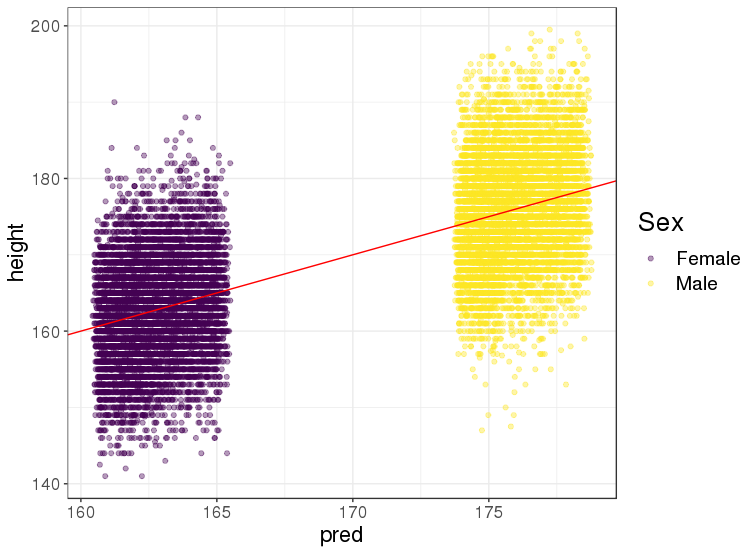In this post, I show some results of predicting height based on DNA mutations. This analysis aims at reproducing the analysis of this paper using my own analysis tools in. I use a new dataset composed of 500,000 adults from UK, and genotyped over hund...

## Fast R functions to get first principal components

August 29, 2018
ByIn this post, I compare different approaches to get first principal components of large matrices in R. Comparison library(bigstatsr) library(tidyverse) Data # Create two matrices, one with some structure, one without n

## Whether to use a data frame in R?

July 19, 2018
By

In this post, I try to show you in which situations using a data frame is appropriate, and in which it’s not. Learn more with the Advanced R book. What is a data frame? A data frame is just a list of vectors of the same length, each vector being a column. This may convince you: str(iris) ## 'data.frame':...

## Why I rarely use apply

July 13, 2018
By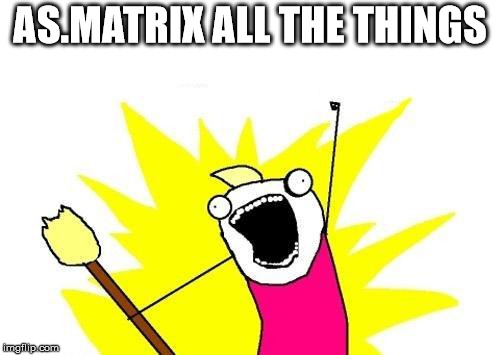In this short post, I talk about why I’m moving away from using function apply. With matrices It’s okay to use apply with a dense matrix, although you can often use an equivalent that is faster. N

## One year as a subscriber to Stack Overflow

July 1, 2018
By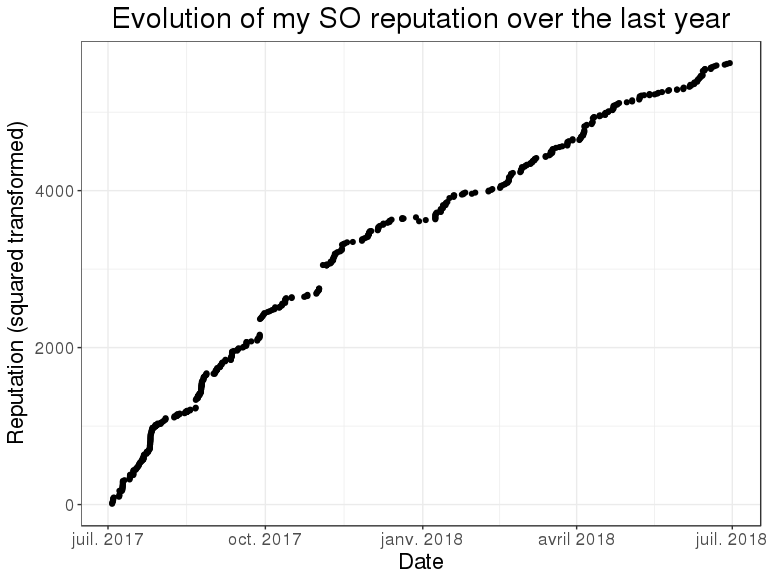In this post, I follow up on a previous post describing how last year in July, I spent one month mostly procrastinating on Stack Overflow (SO). We’re already in July so it’s time to get back to one year of activity on Stack Overflow. Am I still as much active as before? What is my strategy for answering questions...

## Why loops are slow in R

June 10, 2018
By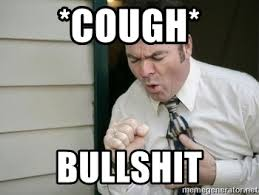In this post, I talk about loops in R, why they can be slow and when it is okay to use them. Don’t grow objects Let us generate a matrix of uniform values (max changing for every column). gen_grow

## Performance: when algorithmics meets mathematics

April 18, 2018
By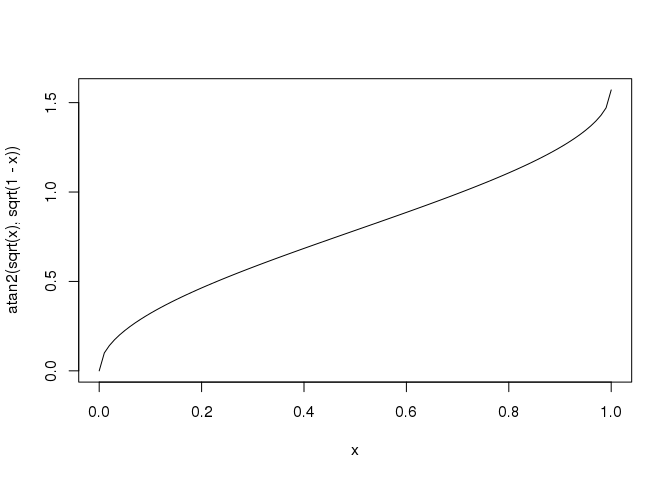In this post, I talk about performance through an efficient algorithm I developed for finding closest points on a map. This algorithm uses both concepts from mathematics and algorithmics. Problem to solve This problem comes from a recent question on StackOverflow. I have two matrices, one is 200K rows long, the other is 20K. For each row (which is...

## Teaching an advanced R course

March 28, 2018
ByIn this post, I come back to my first experience teaching an advanced R course over the past month. Content This course was programmed for 10 sessions (3 hours each) and I initially wanted to talk about the following subjects: R programming and g...